# Carbonic acid

Carbonic acid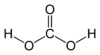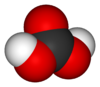Other names Carbon dioxide solution
Identifiers
CAS number [463-79-6]
SMILES C(=O)(O)O
Properties
Molecular formula H2CO3
Molar mass 62.03 g/mol
Density 1.0 g/cm3
(dilute solution)
Solubility in water exists only in solution
Acidity (pKa) 6.36 (see text)
10.25
Except where noted otherwise, data are given for
materials in their standard state
(at 25 °C, 100 kPa)

Carbonic acid (ancient name acid of air or aerial acid) is a weak acid with the formula H2CO3. It is formed in small amounts when carbon dioxide is dissolved in water, and it is usually found only in solution. The salts of carbonic acids are called bicarbonates (or hydrogen carbonates, when the anion is HCO3) and carbonates (when the anion is CO32−).

The presence of carbonic acid in the blood and other body fluids helps control the pH level (acidity) of those fluids.

## Solution in water

Carbon dioxide dissolved in water is in equilibrium with carbonic acid:

CO2 + H2O H2CO3

The equilibrium constant at 25°C is Kh= 1.70×10−3, which indicates that most of the carbon dioxide is not converted into carbonic acid and stays as CO2 molecules. In the absence of a catalyst, the equilibrium is reached quite slowly.

The rate constants are 0.039 s−1 for the forward reaction (CO2 + H2O → H2CO3) and 23 s−1 for the reverse reaction (H2CO3 → CO2 + H2O).

## Acidity of carbonic acid

Carbonic acid has two acidic hydrogens and so two dissociation constants:

H2CO3 HCO3 + H+
Ka1 = 2.5×10−4 mol/L; pKa1 = 3.60 at 25 °C.
HCO3 CO32− + H+
Ka2 = 5.61×10−11 mol/L; pKa2 = 10.25 at 25 °C.

Care must be taken when quoting and using the first dissociation constant of carbonic acid. The value quoted above is correct for the H2CO3 molecule, and shows that it is a stronger acid than acetic acid or formic acid: this might be expected from the influence of the electronegative oxygen substituent. However, carbonic acid only ever exists in solution in equilibrium with carbon dioxide, and so the concentration of H2CO3 is much lower than the concentration of CO2, reducing the measured acidity. The equation may be rewritten as follows (c.f. sulfurous acid):

CO2 + H2O HCO3 + H+
Ka = 4.30×10−7 mol/L; pKa = 6.36.

This figure is quoted as the dissociation constant of carbonic acid, although this is ambiguous: it might better be referred to as the acidity constant of carbon dioxide, as it is particularly useful for calculating the pH of CO2 solutions.

## Role of carbonic acid in blood

Carbonic acid plays a very important role in mammalian blood. It is an intermediate during the transfer of carbon dioxide from the lungs to the blood and vice versa. The conversion of carbon dioxide into carbonic acid is catalyzed by an enzyme (carbonic anhydrase), which enhances the reaction rate by a factor of nearly a billion. Carbonic acid dissociates in the blood (as in other solutions), to produce mainly H+ and HCO3- (bicarbonate) ions. This dissociation is an equilibrium reaction and it helps control the pH level of blood.

## Preparation of pure carbonic acid

For a long time, researchers found it impossible to obtain pure hydrogen bicarbonate (H2CO3) at room temperature (about 20 °C, or about 70 °F). However, in 1991, scientists at NASA's Goddard Space Flight Center (USA) succeeded in making the first pure H2CO3 samples. They did so by exposing a frozen mixture of water and carbon dioxide to high-energy radiation, and then warming to remove the excess water. The carbonic acid that remained was characterized by infrared spectroscopy.

The ability to produce carbonic acid by irradiating a solid mixture of H2O and CO2 has led to suggestions that H2CO3 might be found in outer space, where frozen ices of H2O and CO2 are common, as are cosmic rays and ultraviolet light, to help them react.

The same carbonic acid polymorph (denoted beta-carbonic acid) was prepared by a cryotechnique at the University of Innsbruck: alternating layers of glassy aqueous solutions of bicarbonate and acid were heated in vacuo, which causes protonation of bicarbonate, and the solvent was subsequently removed. A second polymorph (denoted alpha-carbonic acid) was prepared by the same technique at the University of Innsbruck using methanol rather than water as a solvent.

The researchers at Innsbruck reported that pure, water-free carbonic acid is highly stable in the gas phase, with a calculated half-life of 180,000 years. Yet, according to their calculations, the presence of a single molecule of water causes a molecule of carbonic acid to quickly decompose to carbon dioxide and water.

## pH and composition of a carbonic acid solution

At a given temperature, the composition of a pure carbonic acid solution (or of a pure CO2 solution) is completely determined by the partial pressure$p_{CO_2}$ of carbon dioxide above the solution. To calculate this composition, account must be taken of the above equilibria between the three different carbonate forms (H2CO3, HCO3 and CO32−) as well as of the equilibrium between dissolved CO2 and H2CO3 with constant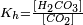$K_h=\frac{[H_2CO_3]}{[CO_2]}$ (see above) and of the following equilibrium between the dissolved CO2 and the gaseous CO2 above the solution:

CO2(gas) ↔ CO2(dissolved) with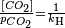$\frac{[CO_2]}{p_{CO_2}}=\frac{1}{k_\mathrm{H}}$ where kH=29.76 atm/(mol/L) at 25°C (Henry constant)

The corresponding equilibrium equations together with the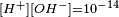$\scriptstyle[H^+][OH^-]=10^{-14}$ relation and the neutrality condition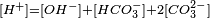$\scriptstyle[H^+]=[OH^-]+[HCO_3^-]+2[CO_3^{2-}]$ result in six equations for the six unknowns [CO2], [H2CO3], [H+], [OH], [HCO3] and [CO32−], showing that the composition of the solution is fully determined by$p_{CO_2}$. The equation obtained for [H+] is a cubic whose numerical solution yields the following values for the pH and the different species concentrations: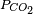$P_{CO_2}$ (atm) pH [CO2] (mol/L) [H2CO3] (mol/L) [HCO3−] (mol/L) [CO32−] (mol/L) 10−8 7.00 3.36 × 10-10 5.71 × 10−13 1.42 × 10−9 7.90 × 10−13 10−6 6.81 3.36 × 10−8 5.71 × 10−11 9.16 × 10−8 3.30 × 10−11 10−4 5.92 3.36 × 10−6 5.71 × 10−9 1.19 × 10−6 5.57 × 10−11 3.5 × 10−4 5.65 1.18 × 10−5 2.00 × 10−8 2.23 × 10−6 5.60 × 10−11 10−3 5.42 3.36 × 10−5 5.71 × 10−8 3.78 × 10−6 5.61 × 10−11 10−2 4.92 3.36 × 10−4 5.71 × 10−7 1.19 × 10−5 5.61 × 10−11 10−1 4.42 3.36 × 10−3 5.71 × 10−6 3.78 × 10−5 5.61 × 10−11 1 3.92 3.36 × 10−2 5.71 × 10−5 1.20 × 10−4 5.61 × 10−11 2.5 3.72 8.40 × 10−2 1.43 × 10−4 1.89 × 10−4 5.61 × 10−11 10 3.42 0.336 5.71 × 10−4 3.78 × 10−4 5.61 × 10−11
• We see that in the total range of pressure, the pH is always largely lower than pKa2 so that the CO32− concentration is always negligible with respect to HCO3 concentration. In fact CO32− play no quantitative role in the present calculation (see remark below).
• For vanishing$p_{CO_2}$, the pH is close to the one of pure water (pH = 7) and the dissolved carbon is essentially in the HCO3 form.
• For normal atmospherics conditions (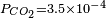$P_{CO_2}=3.5\times 10^{-4}$ atm), we get a slightly acid solution (pH = 5.7) and the dissolved carbon is now essentially in the CO2 form. From this pressure on, [OH] becomes also negligible so that the ionized part of the solution is now an equimolar mixture of H+ and HCO3.
• For a CO2 pressure typical of the one in soda drinks bottles ($P_{CO_2}$ ~ 2.5 atm), we get a relatively acid medium (pH = 3.7) with a high concentration of dissolved CO2. These features are responsible for the sour and sparkling taste of these drinks.
• Between 2.5 and 10 atm, the pH crosses the pKa1 value (3.60) giving a dominant H2CO3 concentration (with respect to HCO3) at high pressures.

As noted above, [CO32−] may be neglected for this specific problem, resulting in the following very precise analytical expression for [H+]: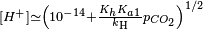$\scriptstyle[H^+] \simeq \left( 10^{-14}+\frac {K_hK_{a1}}{k_\mathrm{H}} p_{CO_2}\right)^{1/2}$